Overview of Light: Reflection and Refraction (Part - 1) (Test)

# Overview of Light: Reflection and Refraction (Part - 1) (Test) - Class 12

 1 Crore+ students have signed up on EduRev. Have you?

https://edurev.in/studytube/Overview-of-Light-Reflection-and-Refraction--Part-/2a48cc71-9e7f-4dc3-b425-64859c519de0_t

1. Speed and Nature of Light

• Light is a form of electromagnetic radiation (radiant energy) that causes the sensation of sight
• In vacuum/air-light travels with a constant speed of 3 x 108 ms-1
• Ordinarily light travels in straight lines but truly speaking light exhibits wave character
• Wavelengths of visible light vary from about 400 nm for violet light to about 700 nm for the red light.

2. The straight-line path along which a light wave travels is called a ray of light. A bundle of rays is called a beam of light.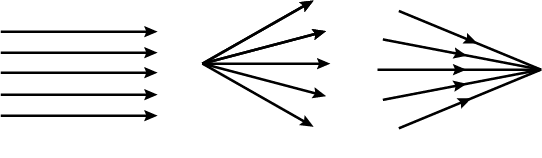(a) Parallel Beam (b) Divergent Beam (c) Convergent Beam

3. When light falls on a surface separating two media, a part of the light is reflected, a part is refracted, and a part is absorbed.

Example:

• A highly polished surface (mirror) mostly reflects the light falling on it.
• A transparent medium refracts most of the light falling on it.
Example: Glass, Water, etc.
• When a number of rays, starting from a point after reflection or refraction, meet or appear to meet at another point, the second point is called the image of the first point

4. Real and Virtual Images

• The image formed is real if the reflected or refracted rays actually meet at a point.
• The image is virtual if the rays do not actually meet, but appear to meet at a point when produced backwards.
• A real image can be obtained on the screen, but a virtual image cannot be obtained on the screen.

5. Two basic laws of reflection of light are:
(a) The angle of incidence (i) is equal to the angle of reflection (r).
(b) The incident ray, the normal to the reflecting surface at the point of incidence, and the reflected ray, all lie in the same plane.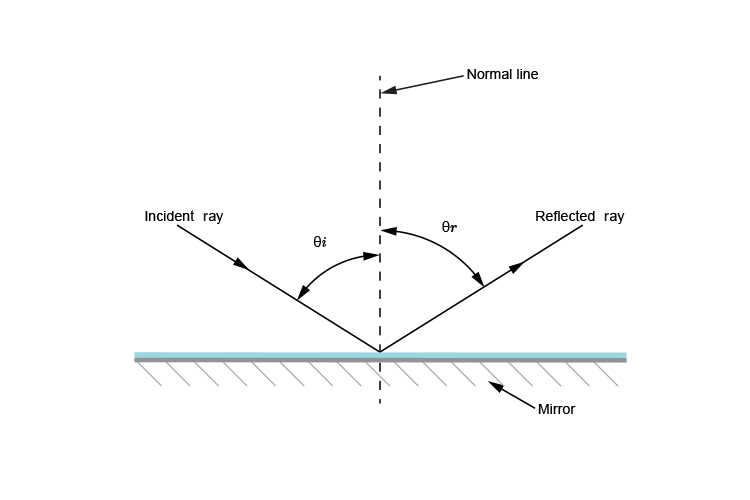6. Image of an extended object formed by a plane mirror has the following characteristics:

• The image formed is virtual and erect.
• The image is of the same size as the object.
• The image is formed as far behind the mirror as the object is in front of it.
• The image is laterally inverted.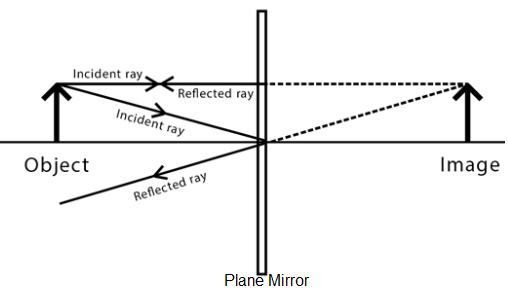An image formed by a plane mirror

7. If the reflecting surface of a mirror is a curved one, it is called a curved mirror.

• Most commonly used curved mirror is the spherical mirror.
•  A spherical mirror is that whose reflecting surface is spherical

8. Spherical Mirrors
(a) Concave Mirror

• A spherical mirror whose reflecting surface is curved inwards is called a concave mirror.

(b) Convex Mirror

• A spherical mirror whose reflecting surface is curved outwards (bulged out) is called a convex mirror. The centre of the reflecting surface of a mirror is called its pole (P).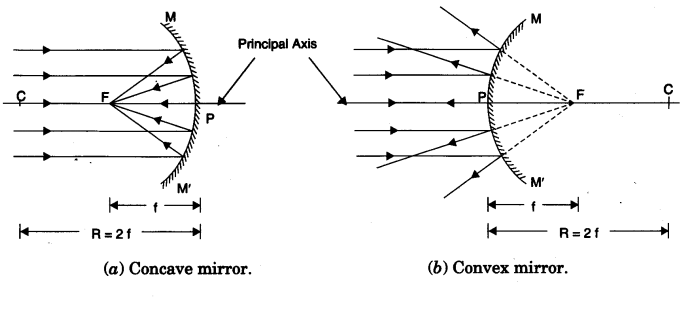Spherical Mirrors

9. Center of Curvature

• The centre of the sphere, the given mirror forms a part of which, is called the centre of curvature (C) of the spherical mirror.
• Centre of curvature of a mirror lies outside its reflecting surface.
• For a concave mirror, the centre of curvature lies in front of it, but for a convex mirror, it lies behind the mirror.
• The distance from the pole to the centre of curvature is known as the radius of curvature of a given mirror. Thus, R = PC

10. Principal Axis and Principal Focus

• A straight line passing through the pole and the centre of curvature of a spherical mirror is called its principal axis
• The principal focus of a spherical mirror is a point on its principal axis where light rays coming parallel to its principal axis, after reflection, actually converge (in case of a concave mirror) or appear to diverge from (in case of a convex mirror).
• Distance from pole to principal focus is called focal length (f) of the given mirror.

11. Focal length of a spherical mirror is half of its radius of curvature. Thus, f = R/2 or 2f

12. The position of the image formed by a spherical mirror can be found by considering any two of the following rays :
(i) The ray incident parallel to the principal axis, after reflection, passes through the principal focus of a concave mirror or appears to pass through the principal focus of a convex mirror.
(ii) A ray passing through the principal focus of a concave mirror or a ray directed towards the principal focus of a convex mirror is reflected parallel to the principal axis of the mirror.
(iii) A ray passing through the centre of curvature in a concave mirror or a ray directed towards the centre of curvature in a convex mirror, after reflection, retraces its path.
(iv) A ray incident obliquely to the principal axis at the pole point is reflected obliquely so that angles subtended by the two rays from the principal axis are equal and in mutually opposite directions.

13. Image Formation by Concave Mirror

• A concave mirror may form either a real or a virtual image depending upon the position of the object.
• The different possibilities are as given in the following table: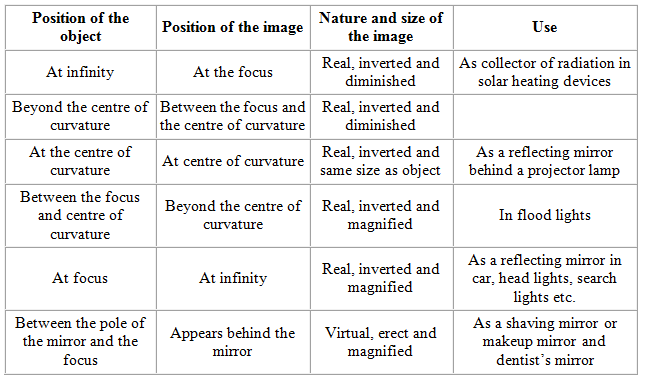14. Image Formation by Convex Mirror

• A convex mirror always forms a virtual, erect and diminished image of a real object.
• When an object is placed at infinity, the point sized image is formed behind the mirror at its principal focus. However, for any other position of the object, the diminished image is formed behind the mirror between its pole P and principal focus F.
Table: Image formed by the convex mirror at different positions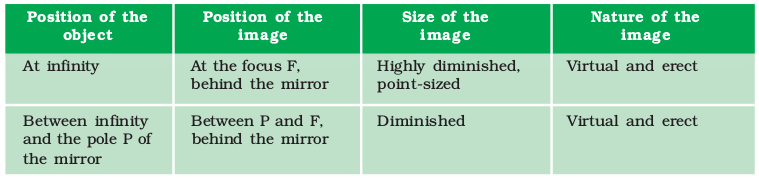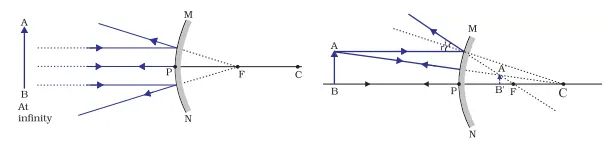Image formation by Convex Mirror

15. While considering reflection from spherical (curved) mirrors, we follow the New Cartesian Sign Convention

According to this convention:
(i) The object is taken on the left of the mirror, i.e., the incident ray strikes the mirror from the left-hand side.
(ii) All the distances parallel to the principal axis are measured from the pole of the mirror.
(iii) Distances in the direction of the incident light are taken positive and in the opposite direction negative. In other words, distances right to the pole are taken positive and distances left to the pole negative.
(iv) The heights measured upwards (i.e., above the principal axis) are taken positive, and the heights measured downwards (below the principal axis) are taken negative.

16. Mirror Formula

• Mirror formula is a relation between the object distance (u), the image distance (v) and the focal length (f) (or the radius of curvature R) of the mirror.
• According to mirror formula: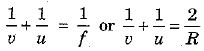• The mirror formula is true for concave and convex mirrors both, irrespective of the fact whether the image formed is real or virtual. However, proper sign for u, v, f and R be applied as per sign convention followed.

17. Magnification

• Magnification (m) of a spherical mirror is defined as the ratio of the height of the image to the height of the object
• It is found that: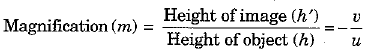• Magnification for the real and inverted image is negative. Magnification for a virtual and erect image is positive.

18. Applications of Concave Mirrors

• Concave mirrors are used in torches, searchlights and headlights of the vehicles to obtain a powerful parallel fight beam.
• Large concave mirrors are used to concentrate sunlight in solar furnaces. Concave mirrors are used as a shaving mirror.
• Dentists use concave mirrors to see a large image of the teeth of patients.

19. Applications of Convex Mirrors

• Convex mirrors are used in vehicles near the driver seat to view traffic coming from behind.
• As convex mirrors form erect and diminished images only, they enable the driver to view a much larger area than would be possible with a plane mirror.

20. Refraction of Light

• Whenever a light ray undergoes oblique refraction from one transparent medium to another, the ray changes its path.
• It is on account of the change in the speed of light on entering the second media

21. Two basic laws of refraction of light are :
(i) The incident ray, the refracted ray and the normal to the separating surface at the point of incidence, all fie in the same plane.
(ii) The ratio of the sine of the angle of incidence (i) to the sine of the angle of refraction (r) is a constant. It is known as Snell’s law

Thus, according to Snell’s law: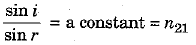Here, n21 is known as the relative refractive index of the second medium with respect to the first medium.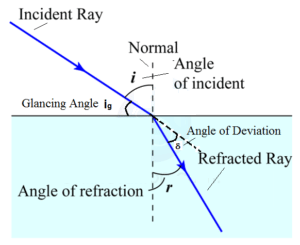22. Refractive Index

• When a light ray is refracted from medium number 1 into medium number 2, the relative refractive index of medium 2 w.r.t. medium 1(n21) is given by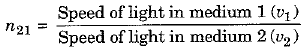• Similarly, the refractive index of medium 1 with respect to medium 2 is given by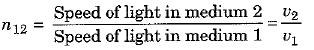• Therefore, we conclude that n21 x n12 = 1
or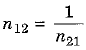The document Overview of Light: Reflection and Refraction (Part - 1) (Test) - Class 12 is a part of Class 12 category.
All you need of Class 12 at this link: Class 12

Track your progress, build streaks, highlight & save important lessons and more!(Scan QR code)

,

,

,

,

,

,

,

,

,

,

,

,

,

,

,

,

,

,

,

,

,

;